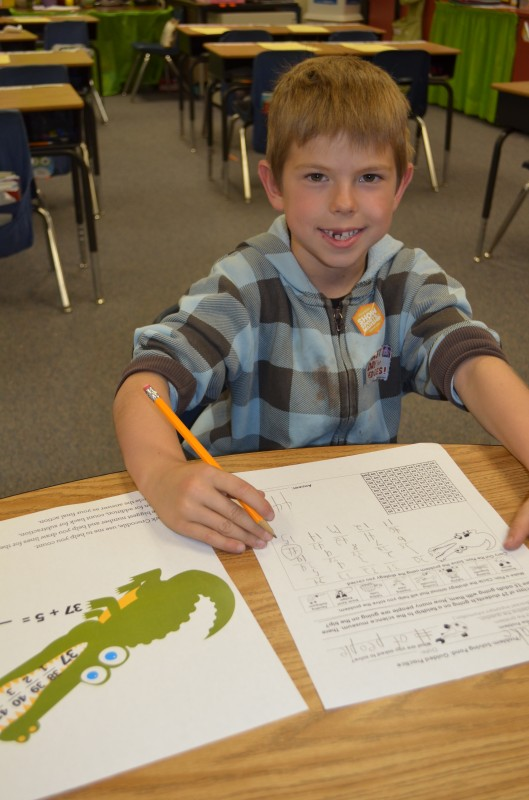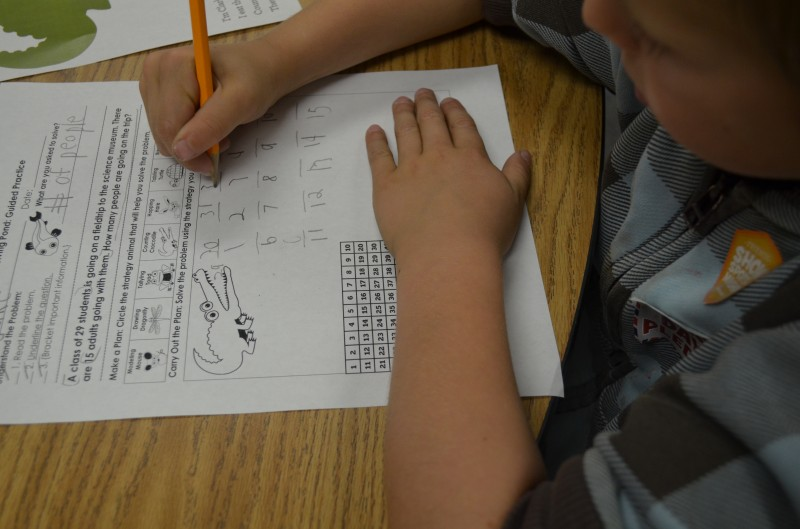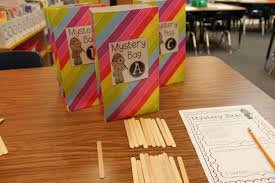### Introducing Clark Counting Crocodile

Start with the largest number, and draw lines for the remaining amount.
Count on for addition, count back for subtraction;Clark Counting Crocodile is the second strategy animal in our Problem-Solving Pond: A Common Core Math Strategy Unit. The Problem-Solving Pond  was created to help teachers overcome Common Core math challenges and employ problem-solving strategies with confidence and fidelity.  Read more about Clark’s strategy below or download the complete unit here.

WHAT is counting?As students build their number sense and fluency, they are able to solve a story problem with counting.  In this strategy, students will learn to count on for addition and count back for subtraction using a 100’s chart for support as needed.

WHY is counting important?Counting is a crucial strategy because it helps students explore the relationships and patterns between numbers. Students need to recognize the order of numbers so they can understand that it is more efficient to start with bigger number. For example, in order to solve “4+27,” they should start counting from 27 and progress to “28, 29, 30, 31” instead of starting from 4 and progressing to 31, because in the latter case, they would have to count much more, increasing the possibility of errors. Proficient counting lays the foundation for number sense and place value.HOW do I teach counting? Explain that Clark helps mathematicians solve problems by counting on to find the total or counting back to find the difference.

• Counting back for subtraction: Students start with the largest number in the problem; they put that number in Clark’s mouth and then draw the number of lines for the second number in the problem (if the second number is 14, students would draw 14 lines). Students then label the lines (below the line) and count back, writing each number above the line. Students circle the answer and write equation below to solidify understanding.

WHEN should I use counting? This strategy isideal for problems that include smaller numbers.  Most teachers use this strategy with K-2 mathematicians, but this is also beneficial for older students as it works for money, multiplication and division (included in unit).

• Create counting bags.  Create counting bags with different numbers of objects in each bag and a set of appropriate number cards. Use common manipulatives such as cubes, beans, tiles for bags. Students also love seasonal items such as conversation hearts, acorns, pumpkins, shamrocks, etc.  Have student count objects in each bag and then select the number card to name that amount. Students can simply place the objects and number card back into the baggie for checking. Mark the bags with letters or shapes for easy checking of student work.• Provide opportunities for strategy exploration. Allow students to build conceptual understanding by having them solve a counting problem in multiple ways. Students can solve the problem starting with the big number first and double-check solution by starting with the smaller number first. This exploration will guide students to recognize that while it is more efficient to start with the larger number in the problem, number order does not affect the sum. Provide templates with larger numbers; this will help students observe that the counting strategy is not efficient for larger numbers.

### Why? The Simple, Yet Essential Question

As teachers, we are programmed to listen for the correct answer and are appeased when we hear it, assuming that students understand and are ready to move on. However, this is a serious misconception as students are missing out on a vital opportunity to explain and justify their thinking.  Asking “Why?” provides critical insight to student understanding as students give the following responses:

• A strong explanation that describes process to class
• An inaccurate explanation that shows a student’s misunderstanding or inability to justify an answer

Besides assessing understanding, asking “Why?” provides students with opportunities to:

• Notice mistake(s) and self-correct the answer
• Reveal mistake(s) or misunderstanding shared by the class
• Take risks and build confidence
• Strengthen communication skills
• Give alternate explanations
• Summarize explanations given by other students

Including this simple question has major consequences: it promotes a language-rich classroom; supports inquiry-based instruction; and builds classroom community.

Asking good follow-up questions can open the door “why-d” to learning.

Download our FREE Essential Questions & Prompts, a sneak peek at our upcoming Common Core Problem Solving Unit.